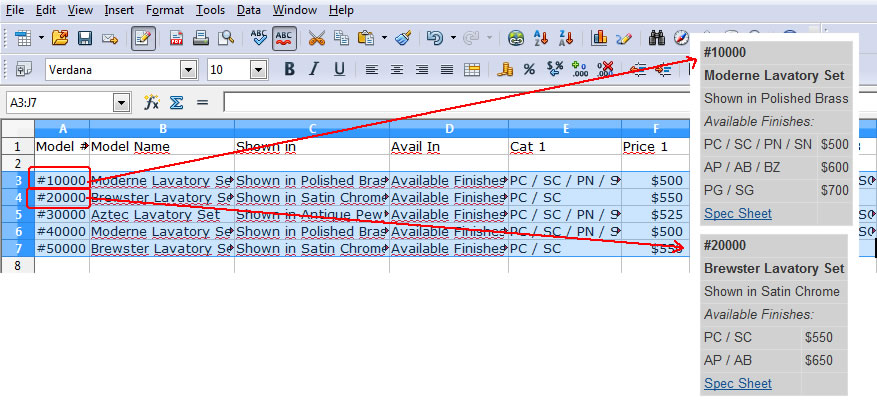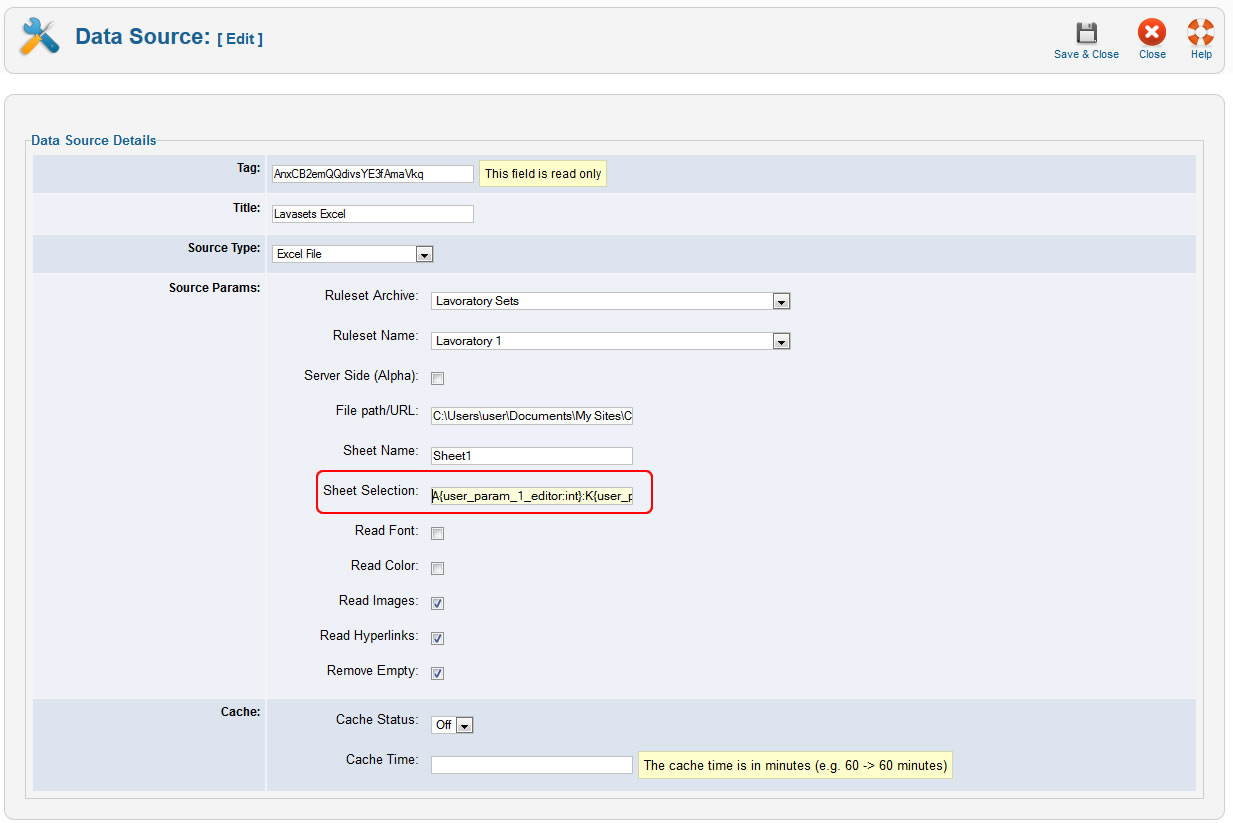# Case study: Using the modification function and data sources with user defined parameters

Tabulizer 4.1 introduced some really cool features that we will demonstrate in this article using a real life example.
Let's start by stating the client's requirements:

1. The product listing is contained in an Excel file, where each row corresponds to a single product, as shown in the figure below:2. The product info needs to be transferred to the site and presented as a table (one table per product).
3. If the product info changes on the Excel file, the associated table needs to be updated as well.Tabulizer can meet all the above requirements using the following techniques:

1. Create a data source that is linked to the Excel file that contains the product listing.
2. Instead of creating one data source for each product, we use one user variable in the definition of the data source, as shown in the figure below:The {user_param_1_editor:int} is a user defined variable of type integer (int) and it will take its value from the editor when we insert the data source. Another alternative could be to get the value from the URL {user_param_1_url:int} or use a combination of these two cases {user_param_1:int} where the default value is given in the editor, but can be overwritten by the value provided in the URL.Now, if user_param_1_editor = 3 the Sheet Selection becomes A3:K3, if user_param_1_editor = 4 the Sheet Selection is A4:K4 and so on.
3. When you insert the data source in the editor's box, apart from selecting the data source we also give the user variable's value, as shown below:The 1:3 value means user_param_1_editor = 3. So 1 is the index of the user variable, while the 3 is it's value. Notice also that the inserted value is MToz which is the base64 encoded value of 1:3. In the optional parameters input box you can specify the values for more than one user defined variables using the semicolon (;) as separator. For instance, 1:3;2:all;3:4.1 will assign user_param_1_editor = 3, user_param_2_editor = all, user_param_3_editor = 4.1.
4. There is one final issue that we need to address. The input array for each product is a single row with many columns.
This does not look very good and instead we would prefer something similar to the table below:
How do we make this transformation? By using a modification function that takes as input the original input table and returns a modified version of it.
The modification function basically can be any PHP function that follows these naming conventions and coding rules:
• Is defined in a separate PHP file that resides in the {joomla root directory}/templates/tabulizer/modify folder.
• The skeleton of this file, assuming that the name of the modification function is "test" is:
`<?php class TabulizerModifyTest {   function modify(&\$rows, \$args = null) {          // do something with the rows   } } ?>`
So, there is a class named TabulizerModifyNameofthefunction that contains a modify method stored in {joomla root directory}/templates/tabulizer/modify/nameofthefunction.php file. The first argument of the modify method is the table rows that are passed by reference, while the second argument is optional function params. There is no return value. Notice also that in the table rows array the row count and the column count start from 1, not 0. So, if you want to see how many rows and columns that input table has, you could write the following snippet:
`      \$num_of_rows = count(\$rows);      \$num_of_columns = count(\$rows);`

In our example, we want to transform the input table array using the following code stored in {joomla root directory}/templates/tabulizer/modify/lav2k2.php file:
`<?php class TabulizerModifyLav2k2 { function modify(&\$rows, \$args = null) {  if (empty(\$rows)) return; \$num_of_columns = count(\$rows); if (\$num_of_columns < 7) return;  \$new_rows = array(); \$row_id = 1; \$new_rows[\$row_id++] = array(1=>\$rows,2=>''); \$new_rows[\$row_id++] = array(1=>\$rows,2=>''); \$new_rows[\$row_id++] = array(1=>\$rows,2=>''); \$new_rows[\$row_id++] = array(1=>\$rows,2=>''); for (\$i=5;\$i<(\$num_of_columns-1);\$i+=2) { \$new_rows[\$row_id++] = array(1=>\$rows[\$i], 2=>\$rows[\$i+1]); } \$new_rows[\$row_id++] = array(1=>'<a href="'.\$rows[\$num_of_columns].'">Spec Sheet</a>',2=>'');  \$rows = \$new_rows;  } } ?>`
5. The modification function is called by the ruleset, that contains some other styling rules. Here is the table rule we added in order to call the modification function named lav2k2:To sum it up, in this real life example we used two new features:

• The data source with user defined variables in order to reduce the total number of required data sources.
• The modification function that can pre-process the table data when no other rule can achieve the same transformation.

For security reason, ruleset archives that contain modification functions will not be imported properly if they contain php files. Therefore, if the ruleset contains a modification function you can either unzip the archive locally and upload the containing folders manually to /template/tabuluzer subfolder, or change the ALLOWED_FILE_TYPES_FOR_ARCHIVE_IMPORTATION constant to:
define('ALLOWED_FILE_TYPES_FOR_ARCHIVE_IMPORTATION','xml,css,jpg,jpeg,gif,png,php');
This constant is defines in {joomla root path}/administrator/components/com_tabulizer/assets/classes/common/defines.php file.